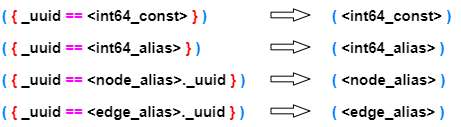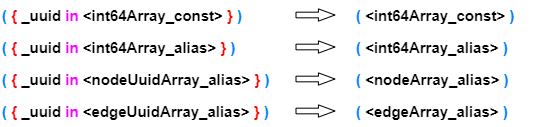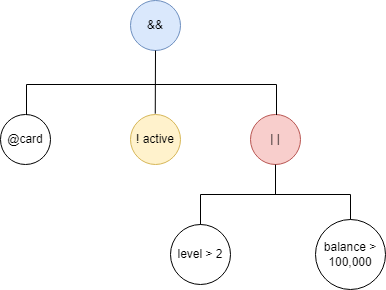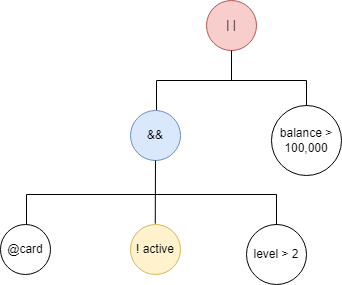# Change Nickname

Current Nickname:
Search
v2.x
v4.0

# Filter

## Expression, Operator, Precedence

Ultipa filter is an expression encapsulated in curly bracket `{ }`, which acquires the property and schema of node/edge by calling the alias. The expression produces `true` if the current node/edge meets the filtering rules. The filtering of node/edge is supported by all Ultipa query operations.

The filter uses two types of operators to determine true or false:

• Conditional operators
Operator Description
@ schema checker
== equal
!= not equal
< less than
> greater than
<= less than or equal
>= greater than or equal
<> between
<=> between or equal
in belong to
nin not belong to
contains contain / full-text search
• Logical operators
Operator Description
&& and
| | or
! not

Numeric operations supported in the filter are:

Operator Description
- subtraction
* multiplication
/ division
% modulo (the remainder of division)

The following table shows the precedence of conditional operators, logical operators and numeric operators (from high to low):

Precedence Operator Catetory
1 ( ), [ ]
2 @ conditional operator
3 ! logical operator
4 *, /, % numeric operator
5 +, - numeric operator
6 >, <, >= , <= , <> , <=>, in, nin, contains conditional operator
7 == , != conditional operator
8 && logical operator
9 | | logical operator

Practically, Ultipa filter also applies to two special scenarios:

• In-step filtering: In template queries, use system alias `prev_n` and `prev_e` to call the property values of the previous node/edge of the current node/edge in the path, and filter them.
• Full-text filtering: In any queries, use the operator `contains` to filter the property values of full-text index.

## Conditional Operator

Judging whether the schema or property of the current node/edge meet the given query rules according to the conditional operators and operands.

Syntax (in the vast majority of cases): {<operand1> <operator> <operand2>}

where `<operand>` must be of the same data type, and it can be constants, node/edge properties, or numeric expression composed of constants and the call of properties.

Call of property can be written as <alias>.<property> or <alias>.@<schema>.<property>, `@schema` can't be included when calling the unique identifier `_id` or `_uuid`.

For properties of number or time data type, conditional operators can be ignored and only operands are contained, when it judges whether the property of the current nodes/edges meets the querying criteria, value `0` represents false, otherwise it means true.

Example: Find 10 people that are not born in leap years

``````find().nodes({birthYear%4})
return nodes{*} limit 10
``````

Analysis: A leap year can be divided by 4.

Example: Find 10 nodes whose "time" (datatime type) is not 0

``````find().nodes({time})
return nodes{*} limit 10
``````

Analysis: The property time is of value 0 means it is "0-0-0 0:0:0". When a datetime property is created for an existent metadata, the default property value is "0-0-0 0:0:0".

### @ (Schema Filter)

Schema filter judges whether the current node/edge belongs to a given schema. Schema filter is often overlaid with property call.

Syntax: {@<schema>}

Example: Find information of 10 bank cards @card

``````find().nodes({@card})
return nodes{*} limit 10
``````

Analysis: Another way of judging the value of schema is `{@ == "<value>"}`, see the description of conditional operator `==`.

### == (Equal)

Syntax: {<operand1> == <operand2>}

Data type of the operand: string, number, time

Abbreviated form: when judging whether the UUID of the current node/edge equals an integer or the UUID of a certain node/edge alias, the filter can be abbreviated as the integer or the node/edge alias without the curly bracketExample: Find information of 10 bank cards

``````find().nodes({@ == "card"})
return nodes{*} limit 10
``````

Example: Find 10 nodes at level 1

``````find().nodes({level == 1})
return nodes{*} limit 10
``````

Example: Find 10 bank cards at level 1

``````find().nodes({@card.level == 1})
return nodes{*} limit 10
``````

Example: Find 1-hop neighbors of node UUID = 1, then find 1-hop neighbors of these neighbors

``````khop().src({_uuid == 1}).depth(1) as neighbor1
khop().src({_uuid == neighbor1._uuid}).depth(1) as neighbor2
return distinct(neighbor2)
``````

Example: Simplify the UQL in the above example

``````khop().src(1).depth(1) as neighbor1
khop().src(neighbor1).depth(1) as neighbor2
return distinct(neighbor2)
``````

### != (Not Equal)

Syntax: {<operand1> != <operand2>}

Data type of the operand: string, number, time

Example: Find 10 bank cards with level not 1

``````find().nodes({@card.level != 1})
return nodes{*} limit 10
``````

Example: Find bank cards of account U001 with level not 1

``````n({_id == "U001"}).re().n({@card.level != 1} as cards)
return cards
``````

### < (Less Than)

Syntax: {<operand1> < <operand2>}

Data type of the operand: string, number, time

Example: Count cards with balance below 10

``````find().nodes({@card.balance < 10})
return count(nodes)
``````

### > (Greater Than)

Syntax: {<operand1> > <operand2>}

Data type of the operand: string, number, time

Example: Count cards with balance beyond 10,000

``````find().nodes({@card.balance > 10000})
return count(nodes)
``````

### <= (Less Than or Equal)

Syntax: {<operand1> <= <operand2>}

Data type of the operand: string, number, time

Example: Count cards at level 2 or less

``````find().nodes({@card.level <= 2})
return count(nodes)
``````

### >= (Greater Than or Equal)

Syntax: {<operand1> >= <operand2>}

Data type of the operand: string, number, time

Example: Count cards at level 3 or greater

``````find().nodes({@card.level >= 3})
return count(nodes)
``````

### <> (Between)

Syntax: {<operand1> <> [<operand2>,<operand3>]}, note that <operand2> must be less than <operand3>

Data type of the operand: string, number, time

Example: Count transactions happened between "2021-01-01 00:00:00.000000" and "2021-01-02 00:00:00.000000"

``````find().edges({@transaction.time <> ["2021-01-01 00:00:00.000000","2021-01-02 00:00:00.000000"]})
return count(edges)
``````

Example: Count edges happened between "2021-01-01 00:00:00.000000" and "2021-01-02 00:00:00.000000"

``````find().edges({time <> ["2021-01-01 00:00:00.000000","2021-01-02 00:00:00.000000"]})
return count(edges)
``````

### <=> (Between or Equal)

Syntax: {<operand1> < = > [<operand2>,<operand3>]}, note that <operand2> must be less than <operand3>

Data type of the operand: string, number, time

Example: Count cards at level 2 to 4

``````find().nodes({@card.level <=> [2,4]})
return count(nodes)
``````

### in (Belong to)

Syntax: {<operand1> in [<operand2>,<operand3>, ...]}

Data type of the operand: string, number, time

Abbreviated form: when judging whether the UUID of the current node/edge belongs to an array of integers or an array of UUIDs of a node/edge array alias, the filter can be abbreviated as the array of integers or the node/edge array alias without the curly bracketExample: Find nodes with UUID = 1 or 2 or 3

``````find().nodes({_uuid in [1,2,3]})
return nodes{*}
``````

Example: Simplify the UQL in the above example

``````find().nodes([1,2,3])
return nodes{*}
``````

Example: Find students from Bejing, Shanghai or Tianjin

``````find().nodes({@student.city in ["Bejing", "Shanghai", "Tianjin"]})
return nodes{*}
``````

Example: Find 1-hop neighbors of node UUID = 1, then find 1-hop neighbors of these neighbors

``````khop().src(1).depth(1) as neighbor1
with collect(neighbor1._uuid) as uuidArray
n({_uuid in uuidArray}).e().n(as neighbor2)
return distinct(neighbor2)
``````

Example: Simplify the UQL of the above example

``````khop().src(1).depth(1) as neighbor1
with collect(neighbor1._uuid) as uuidArray
n(uuidArray).e().n(as neighbor2)
return distinct(neighbor2)

// or

khop().src(1).depth(1) as neighbor1
with collect(neighbor1) as nodeArray
n(nodeArray).e().n(as neighbor2)
return distinct(neighbor2)
``````

### nin (Not Belong to)

Syntax: {<operand1> nin [<operand2>,<operand3>, ...]}

Data type of the operand: string, number, time

Example is omitted.

### contains (Contain)

Syntax: {<string> contains "<substring>"}

where `<substring>` should use backslash `\` as the prefix if has English double quotation marks.

Example: Find accounts whose email address contains "@ultipa.com"

``````find().nodes({email contains "@ultipa.com"})
return nodes
``````

### contains (Full-text Search)

Syntax: {~<fulltext> contains "<keyword1> <keyword2> ..."}

where space is used to separate multiple `<keyword>`, and should use backslash `\` as the prefix if has English double quotation marks in a `<keyword>`; `<keyword>` used for fuzzy matching should end with asterisk `*`.

Example: Search products that contain keywords "graph" and "database" by the full-text index prodDesc

``````find().nodes({~prodDesc contains "graph database"})
return nodes
``````

For more examples of full-text index, please refer to Full-text Index under chapter Index.

## Logic Operator

Logic operator combines multiple sub-conditions into a more complex logical judgement basis.

Syntax: {<sub_condition1> <operator1> <sub_condition2> <operator2> ...<sub_conditionN>}

### && (And)

Example: Similar to the example of Between or Equal operator `<=>`, count cards at level 2 to 4

``````find().nodes({@card && level <=> [2,4]})
return count(nodes)
``````

Note: The combination of schema filter and property filter `{@<schema>.<property> <operator> <value>}` is equivalent to a logic tree which organizes `@<schema>` and `<property> <operator> <value>` the two sub-conditions by `&&`.

Example: Find 10 cards with at level greater than 2 and balance beyond 100,000

``````find().nodes({@card && balance > 100000 && level > 2})
return nodes{*} limit 10
``````

### || (Or)

Example: Find 10 cards at level greater than 2 or balance beyond 100,000

``````find().nodes({@card && (balance > 100000 || level > 2)})
return nodes{*} limit 10
``````

Note: `||` has lower precedence than `&&`, but the parenthesis `( )` can raise its priority.

### ! (Not)

Example: Find 10 nodes that are not bank accounts

``````find().nodes({!@account})
return nodes{*} limit 10
``````

Example: Find 10 inactive accounts, node property active is 1 when the account is active and 0 when the account is inactive

``````find().nodes({@account && !active})
return nodes{*} limit 10
``````

### Comprehensive Applications (Logic Tree)

Example: Write the UQL of the logic tree as shown below, find 10 inactive cards at level greater than 2 or balance beyond 100,000``````find().nodes({@card && !active && (level > 2 || balance > 100000)})
return nodes{*} limit 10
``````

Example: Write the UQL of the logic tree as shown below, which removes the parenthesis in the previous example (find 10 nodes that are either inactive cards at level greater than 2, or cards with balance greater than 100,000)``````find().nodes({@card && !active && level > 2 || balance > 100000})
return nodes{*} limit 10
``````